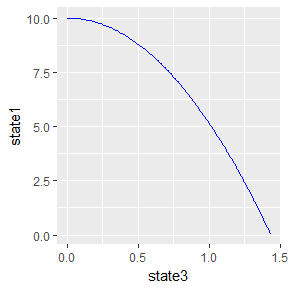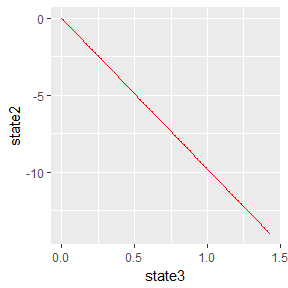# Falling Particle ODE

## The FallingParticleODE class using the Euler ODE solver

Because this is free fall of a particle in one-dimension, for vertical motion, we use Newton’s second law:

$m \frac{d^2y} {dt^2} = F$ and we know that the gravitational force is: $F = -mg$ Therefore: $m \frac{d^2y} {dt^2} = -g$ That expressing as first-order differential equations: $\frac {dy}{dt} = v \\ \frac {dv}{dt} = -g$

($$y$$), we define the numerical state equations as:

state  x
state  v
state  t

From the equations of motion for a falling particle, the derivatives are: $\dot s_1 = s_2 \\ \dot s_2 = -g \\ \dot s_3 = 1$ which is equivalent of writing this as the rate in the code: $r_1 = r_2 \\ r_2 = -g \\ r_3 = 1$

## Build the FallingParticleODE class

We don’t indicate the ODE solver at this time. That is done in the application in the next section.

library(rODE)

# This code can also be found in the examples folder under this name:
# FallingParticleODE.R
#

setClass("FallingParticleODE", slots = c(
g = "numeric"
),
prototype = prototype(
g = 9.8
),
contains = c("ODE")
)

setMethod("initialize", "FallingParticleODE", function(.Object, ...) {
.Object@state <- vector("numeric", 3)
return(.Object)
})

setMethod("getState", "FallingParticleODE", function(object, ...) {
# Gets the state variables.
return(object@state)
})

setMethod("getRate", "FallingParticleODE", function(object, state, ...) {
# Gets the rate of change using the argument's state variables.
object@rate <- state
object@rate <- - object@g
object@rate <- 1

object@rate
})

# constructor
FallingParticleODE <- function(y, v) {
.FallingParticleODE <- new("FallingParticleODE")
.FallingParticleODE@state <- y
.FallingParticleODE@state <- v
.FallingParticleODE@state <- 0
.FallingParticleODE
}
##  "initialize"
##  "getState"
##  "getRate"

## Run the application FallingParticleODEApp

# This code can also be found in the examples folder under this name:
#
# FallingParticleODEApp.R
#
#
FallingParticleODEApp <- function(verbose = FALSE) {
library(ggplot2)

# load the R class that sets up the solver for this application

initial_y <- 10   # initial y position
initial_v <- 0    # initial x position
dt        <- 0.01 # delta time for step

ball <- FallingParticleODE(initial_y, initial_v)

solver <- Euler(ball)
solver <- setStepSize(solver, dt)

rowVector <- vector("list")
i <- 1
# stop loop when the ball hits the ground
while (ball@state >= 0) {
rowVector[[i]] <- list(state1 = ball@state,
state2 = ball@state,
state3 = ball@state)
solver <- step(solver)
ball <- solver@ode
if (verbose) {
cat(sprintf("%12f %12f ",  ball@state, ball@rate ))
cat(sprintf("%12f %12f ",  ball@state, ball@rate ))
cat(sprintf("%12f %12f\n", ball@state, ball@rate ))
}
i <- i + 1
}

DT <- data.table::rbindlist(rowVector)
print(ggplot(DT, aes(x = state3, y = state1)) + geom_line(col = "blue"))
print(ggplot(DT, aes(x = state3, y = state2)) + geom_line(col = "red"))
}

FallingParticleODEApp()